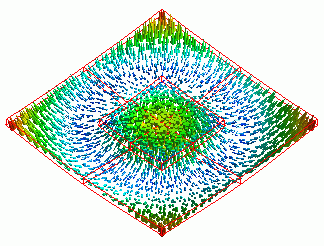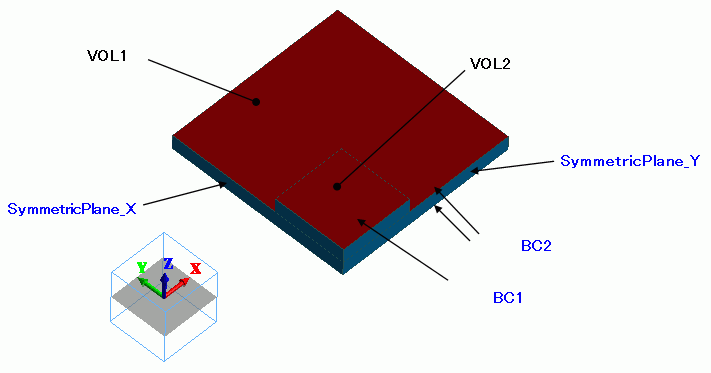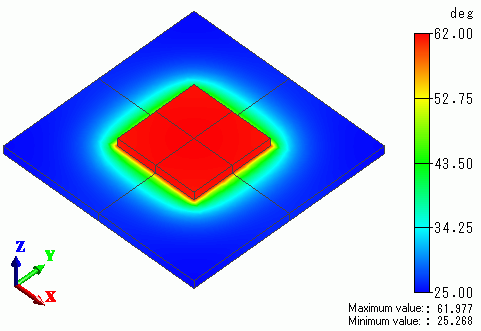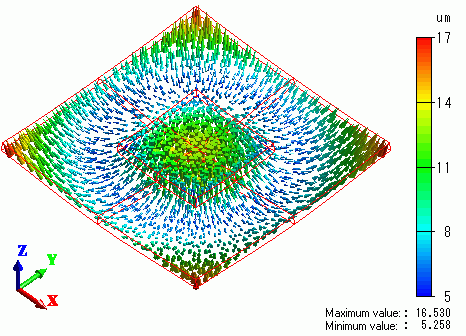﻿ Model with Reflective SymmetryExamples | Product | Murata Software Co., Ltd.# Example7Model with Reflective Symmetry### General

• A quarter model of Exercise 2 is analyzed with reflective symmetry applied.
The figure above is obtained with [Full Model].

• The reflective symmetry is used as boundary condition.

• The temperature gradient caused by the heating chip is calculated by thermal analysis [Watt].
The result is passed on to Galileo as a thermal load.

• The deformation, the displacement and the mechanical stress are solved.

• Unless specified in the list below, the default conditions will be applied.

### Analysis Space

 Item Settings Analysis Space 3D Model unit mm

### Analysis Conditions

Select Thermal analysis and Mechanical stress analysis.

 Item Settings Solver Thermal Analysis [Watt] Mechanical Stress Analysis [Galileo] Thermal-Analysis Type Steady-State Analysis Options N/A *

* “Thermal Load” is selected by default for the thermal load-mechanical stress coupled analysis.

The Step/Thermal Load tab is set as follows.

 Tabs Setting Item Settings Step/Thermal Load * Reference temperature 25[deg]

* The reached temperatures come from the thermal analysis.

### Model

This is a quarter model of Exercise 2.

The planes of symmetry are YX and ZX planes.### Body Attributes and Materials

 Body Number/Type Body Attribute Name Material Name 0/Solid VOL1 006_Glass_epoxy * 1/Solid VOL2 001_Alumina *

* Available from the Material DB

As for the heat source of VOL2

enter 0.25[W] which is a quarter of the original model.

 Body Attribute Name Tab Setting VOL2 Heat Source 0.25[W]

### Boundary Conditions

Set reflective symmetry on the applicable topologies.

 Boundary Condition Name/Topology Tab Boundary Condition Type Settings SymmetricPlane_X/Face Symmetry/Continuity Symmetry Reflective SymmetricPlane_Y/Face Symmetry/Continuity Symmetry Reflective

The heat transfer coefficients for the forced convection are calculated as follows. See [Heat Transfer/Ambient Radiation] for more information.

h = 3.86 x (V/L)0.5xC [W/m2/deg]

where

Air flow V=1[m/s]

Top and bottom faces of the substrate (VOL1): Characteristic length L=0.05, C=1 -> h=17.26
Top face of the heat source (VOL2): Characteristic length L=0.02, L’=0.015, C=1 * -> h=27.3

*

The thickness (d) of the speed boundary layer at the edges of the heat source is given by

d=0.0182x(L’/V)0.5= 2.3[mm]

This is close enough to the thickness of heat source, so we set C=1.

 Boundary Condition Name/Topology Tab Boundary Condition Type Settings BC1/Face Thermal Heat Transfer/Ambient Radiation Heat transfer coefficient: 17.26[W/m2/deg] Room temperature: 25[deg] BC2/Face Thermal Heat Transfer/Ambient Radiation Heat transfer coefficient: 27.3[W/m2/deg] Room temperature: 25[deg]

Thermal analysis is performed based on the boundary conditions below. The resulting temperature distribution is forwarded to mechanical stress analysis.

### Results

The temperature distribution as a result of Watt is shown below.The next figure shows the vectors of displacement as a result of Galileo following Watt.They are quite similar to the results of Exercise 2.

﻿